# NCERT Solutions for Class 6 Maths Chapter 8

## NCERT Solutions for Class 6 Maths Chapter 8 - Free PDF Download

Understanding decimals when studying Class 6 Maths Chapter 8 Decimals Solutions is necessary as it is an integral part of the student’s syllabus. This chapter mostly focuses on the areas of exactly how decimals work. It begins by providing a student with a short introduction of exactly what are decimals and how they are formed. It then proceeds to elaborate about tenth and hundredth decimals and their significance in calculations.

In this chapter, you also learn about the usage of decimals in different segments of calculation in mathematics. Understanding the subject matter is necessary for students to score well in this chapter.

Every NCERT Solution is provided to make the study simple and interesting on Vedantu. You can also download NCERT Solution for Class 6 Science to score more in exams.

Do you need help with your Homework? Are you preparing for Exams?
Study without Internet (Offline)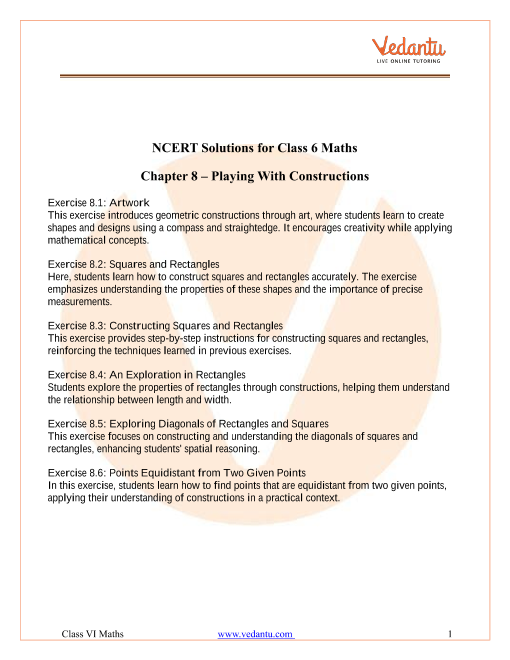Book your Free Demo session
Get a flavour of LIVE classes here at Vedantu## Access NCERT Solutions for Class 6 Maths Chapter 8 - Decimals

Q1: Write the following numbers in the given table.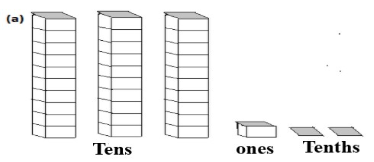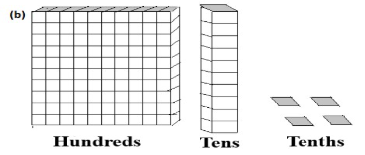Row Hundreds$$\left( {100} \right)$$ Tens$$\left( {10} \right)$$ Ones$$\left( 1 \right)$$ Tenths$$\left( {\dfrac{1}{{10}}} \right)$$ (a) (b)

Ans:

 Row Hundreds$$\left( {100} \right)$$ Tens$$\left( {10} \right)$$ Ones$$\left( 1 \right)$$ Tenths$$\left( {\dfrac{1}{{10}}} \right)$$ (a) $$0$$ $$3$$ $$1$$ $$2$$ (b) $$1$$ $$1$$ $$0$$ $$4$$

Q2. Write the following decimals in the place value table:

1. $$19.4$$

2. $$0.3$$

3. $$10.6$$

4. $$205.9$$

Ans:

 Decimals Hundreds Tens Ones Tenths $$19.4$$ $$0$$ $$1$$ $$9$$ $$4$$ $$0.3$$ $$0$$ $$0$$ $$0$$ $$3$$ $$10.6$$ $$0$$ $$1$$ $$0$$ $$6$$ $$205.9$$ $$2$$ $$0$$ $$5$$ $$9$$

Q3. Write each of the following as decimals:

1. Seven-tenths

2. Two tens and nine-tenths

3. One hundred and two-ones

4. Six hundred point eight

Ans:

a) The decimal form of seven-tenths is

$$\dfrac{7}{{10}} = 0.7$$

b) The decimal form of two tens and nine-tenths is

$$2 \times 10 + \dfrac{9}{{10}} = 20 + 0.9 = 20.9$$

c) The decimal form of one hundred and two-ones is

$$100 + 2 \times 1 = 102.0$$

d) The decimal form of six hundred point eight is $$600.8$$.

Q4. Write each of the following as decimals:

(a)  $$\dfrac{5}{{10}}$$

(b) $$3 + \dfrac{7}{{10}}$$

(c) $$200 + 60 + 5 + \dfrac{1}{{10}}$$

(d) $$70 + \dfrac{8}{{10}}$$

(e) $$\dfrac{{88}}{{10}}$$

(f) $$4\dfrac{2}{{10}}$$

(g)  $$\dfrac{3}{2}$$

(h) $$\dfrac{2}{5}$$

(i) $$\dfrac{{12}}{5}$$

(j) $$3\dfrac{3}{5}$$

(k) $$4\dfrac{1}{2}$$

Ans:

1.  $$\dfrac{5}{{10}} = 0.5$$

2. $$3 + \dfrac{7}{{10}}$$

$$\Rightarrow \,3 + 0.7 = 3.7$$

1. $$200 + 60 + 5 + \dfrac{1}{{10}}$$

$$\Rightarrow \,200 + 60 + 5 + 0.1 = 265.1$$

1. $$70 + \dfrac{8}{{10}}$$

$$\Rightarrow \,70 + 0.8 = 7.8$$

1. $$\dfrac{{88}}{{10}} = 8.8$$

2. $$4\dfrac{2}{{10}}$$

$$\Rightarrow \,\,\,\dfrac{{42}}{{10}} = 4.2$$

1. $$\dfrac{3}{2}$$

$$\Rightarrow \,\,\dfrac{3}{2} = \dfrac{{2 + 1}}{2}$$

$$\Rightarrow \,\,\dfrac{2}{2} + \dfrac{1}{2}$$

$$\Rightarrow \,\,1 + 0.5 = 1.5$$

1. $$\dfrac{2}{5} = 0.4$$

2. $$\dfrac{{12}}{5}$$

$$\Rightarrow \,\,\dfrac{{12}}{5} = \dfrac{{10 + 2}}{5}$$

$$\Rightarrow \,\,\dfrac{{10}}{5} + \dfrac{2}{5}$$

$$\Rightarrow \,\,2 + 0.4 = 2.4$$

1. $$3\dfrac{3}{5}$$

$$\Rightarrow \,\,\dfrac{{18}}{5}$$

$$\Rightarrow \,\,\dfrac{{18}}{5} = \dfrac{{15 + 3}}{5}$$

$$\Rightarrow \,\,\dfrac{{15}}{5} + \dfrac{3}{5}$$

$$\Rightarrow \,\,3 + 0.6 = 3.6$$

(k) $$4\dfrac{1}{2}$$

$$\Rightarrow \,\,\dfrac{9}{2}$$

$$\Rightarrow \,\,\dfrac{9}{2} = \dfrac{{8 + 1}}{2}$$

$$\Rightarrow \,\,\dfrac{8}{2} + \dfrac{1}{2}$$

$$\Rightarrow \,\,4 + 0.5 = 4.5$$

Q5. Write the following decimals as a fraction. Reduce the fractions to lowest terms:

1. $$0.6$$

2. $$2.5$$

3. $$1.0$$

4. $$3.8$$

(e) $$13.7$$

(f) $$21.2$$

(g) $$6.4$$

Ans:

1. $$0.6$$

$$\Rightarrow \,\,\dfrac{6}{{10}}$$

Divide both number and denominator by 3.

$$\Rightarrow \,\,\dfrac{3}{5}$$

$$\therefore 0.6 = \,\dfrac{3}{5}$$

1. $$2.5$$

$$\Rightarrow \dfrac{{25}}{{10}}$$

Divide both number and denominator by 5.

$$\Rightarrow \dfrac{5}{2}$$

$$\therefore 2.5 = \,\dfrac{5}{2}$$

1. $$1.0$$

$$\Rightarrow \,\,\dfrac{1}{1}$$

$$\therefore \,\,1.0 = \,1$$

1. $$3.8$$

$$\Rightarrow \,\,\dfrac{{38}}{{10}}$$

Divide both number and denominator by 2.

$$\Rightarrow \,\,\dfrac{{19}}{5}$$

$$\therefore \,\,3.8 = \,\dfrac{{19}}{5}$$

1. $$13.7$$

$$\Rightarrow \,\,\dfrac{{137}}{{10}}$$

$$\therefore \,\,13.7 = \,\dfrac{{137}}{{10}}$$

1. $$21.2$$

$$\Rightarrow \,\,\dfrac{{212}}{{10}}$$

Divide both number and denominator by 2.

$$\Rightarrow \,\,\dfrac{{106}}{5}$$

$$\therefore \,\,21.2 = \,\dfrac{{106}}{5}$$

1. $$6.4$$

$$\Rightarrow \,\,\dfrac{{64}}{{10}}$$

Divide both number and denominator by 2.

$$\Rightarrow \,\,\dfrac{{32}}{5}$$

$$\therefore \,\,6.4 = \,\dfrac{{32}}{5}$$

Q6. Express the following as cm using decimals:

(a) 2 mm

(b) 30 mm

(c) 116 mm

(d) 4 cm 2 mm

(e) 162 mm

(f) 83 mm

Ans:

Remember

$$1\,cm = 10\,mm$$

$$1 mm = \dfrac{1}{{10}}\,cm$$

1. $$2 mm = \dfrac{2}{{10}}\,cm$$

$$\therefore \,\,2 mm = 0.2\,cm$$

1. $$30 mm = \dfrac{{30}}{{10}}\,cm$$

$$\therefore \,\,30mm = 3\,cm$$

1. $$116 mm = \dfrac{{116}}{{10}}\,cm$$

$$\therefore \,\,116 mm = 11.6\,cm$$

1. $$4\,cm 2 mm = \left[ {4 + \dfrac{2}{{10}}} \right]\,cm$$

$$\therefore \,\,4\,cm 2 mm = 4.2\,\,cm$$

1. $$162 mm = \dfrac{{162}}{{10}}\,cm$$

$$\therefore \,\,162 mm = 16.2\,cm$$

1. $$83 mm = \dfrac{{83}}{{10}}\,cm$$

$$\therefore \,\,83 mm = 8.3\,cm$$

Q7. Between which two whole numbers on the number line are the given numbers lie? Which of these whole numbers is nearer the number?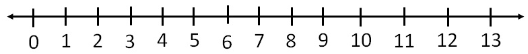(a) $$0.8$$ (b) $$5.1$$ (c) $$2.6$$ (d) $$6.4$$

(e) $$9.1$$ (f) $$4.9$$

Ans:

1. $$0.8$$ lies between $$0$$ and $$1$$

$$\therefore \,\,0.8$$ is nearer to $$1$$.

1. $$5.1$$ lies between $$5$$ and $$6$$

$$\therefore \,\,5.1$$ is nearer to $$5$$.

1. $$2.6$$ lies between $$2$$ and $$3$$

$$\therefore \,\,2.6$$ is nearer to $$3$$.

1. $$6.4$$ lies between $$6$$ and $$7$$

$$\therefore \,\,6.4$$ is nearer to $$6$$.

1. $$9.1$$ lies between $$9$$ and $$10$$

$$\therefore \,\,9.1$$ is nearer to $$9$$.

1. $$4.9$$ lies between $$4$$ and $$5$$

$$\therefore \,\,4.9$$ is nearer to $$5$$.

Q8. Show the following numbers on the number line.

(a) $$0.2$$

(b) $$1.9$$

(c) $$1.1$$

(d) $$2.5$$

Ans:

1. Decimal $$0.2$$ lies between the points $$0$$ and $$1$$ on the number line, such that the space between $$0$$ and $$1$$ is divided into $$10$$ equal parts. Therefore, each equal part will be equal to one-tenth $$\left( {\dfrac{1}{{10}} = 0.1} \right)$$. So, $$0.2$$ is the second point between $$0$$ and $$1$$.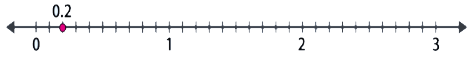1. Decimal $$1.9$$ lies between the points $$1$$ and $$2$$ on the number line, such that the space between $$1$$ and $$2$$ is divided into $$10$$ equal parts. Therefore, each equal part will be equal to one-tenth $$\left( {\dfrac{1}{{10}} = 0.1} \right)$$. So, $$1.9$$ is the ninth point between $$1$$ and $$2$$.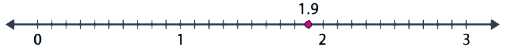1. Decimal $$1.1$$ lies between the points $$1$$ and $$2$$ on the number line, such that the space between $$1$$ and $$2$$ is divided into $$10$$ equal parts. Therefore, each equal part will be equal to one-tenth $$\left( {\dfrac{1}{{10}} = 0.1} \right)$$. So, $$1.1$$ is the first point between $$1$$ and $$2$$.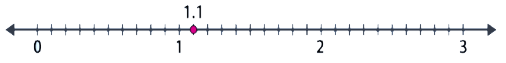1. Decimal $$2.5$$ lies between the points $$2$$ and $$5$$ on the number line, such that the space between $$2$$ and $$5$$ is divided into $$10$$ equal parts. Therefore, each equal part will be equal to one-tenth $$\left( {\dfrac{1}{{10}} = 0.1} \right)$$. So, $$1.1$$ is the fifth point between $$2$$ and $$5$$.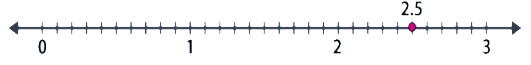Q9. Write the decimal number represented by the points A, B, C, and D on the given number line.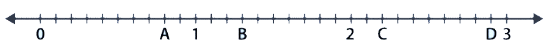Ans:

In a given number line each point will be equal to one-tenth $$\left( {\dfrac{1}{{10}} = 0.1} \right)$$.

1. Point A lies in the eighth point between $$0$$ and $$1$$. So, point A represents $$0.8$$

2. Point B lies in the third point between $$1$$ and $$2$$. So, point B represents $$1.3$$

3. Point C lies in the second point between $$2$$ and $$3$$. So, point C represents $$2.2$$

4. Point D lies in the ninth point between $$2$$ and $$3$$. So, point D represents $$2.9$$

Q10.

1. The length of Ramesh’s notebook is 9 cm 5 mm. What will be its length in cm?

2. The length of a young gram plant is 65 mm. Express its length in cm.

Ans:

1. Given, the length of Ramesh notebook is $$9\,cm 5 mm$$

The length of his notebook in cm is

$$\Rightarrow \,\,9 + \dfrac{5}{{10}}$$

$$\Rightarrow \,\,9 + 0.5 = 9.5\,cm$$

$$\therefore$$ The length of Ramesh notebook is $$9.5\,cm$$.

1. Given, the length of a young gram plant is $$65 mm$$

The length of a young gram plant in cm is

$$\Rightarrow \,\,\dfrac{{65}}{{10}}$$

$$\Rightarrow \,\,6.5\,cm$$

$$\therefore$$ The length of a young gram plant is $$6.5\,cm$$.

Exercise- 8.2

Q1. Complete the table with the help of these boxes and use decimals to write the number.

(a)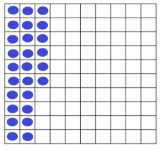(b)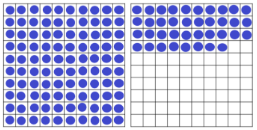(c)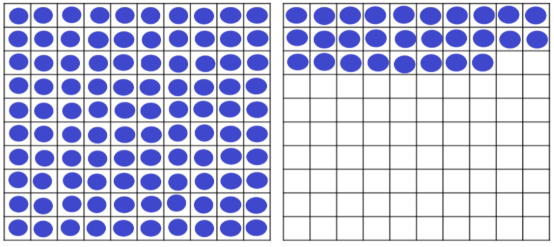Ones Tenths Hundredths Numbers (a) (b) (c)

Ans: The balls present in the rectangle boxes will represent the number. Dividing the total number of balls in a square box by the total number of squares in the square box will give the decimal number. Suppose if we have another square that number should be added.

 Ones Tenths Hundredths Numbers (a) $$0$$ $$2$$ $$6$$ $$0.26$$ (b) $$1$$ $$3$$ $$8$$ $$1.38$$ (c) $$1$$ $$2$$ $$8$$ $$1.28$$

Q2. Write the numbers given in the following place value table in the decimal form:

 Hundreds$$100$$ Tens$$10$$ Ones$$1$$ Tenths$$\dfrac{1}{{10}}$$ Hundredths$$\dfrac{1}{{100}}$$ Thousandths$$\dfrac{1}{{1000}}$$ (a) $$0$$ $$0$$ $$3$$ $$2$$ $$5$$ $$0$$ (b) $$1$$ $$0$$ $$2$$ $$6$$ $$3$$ $$0$$ (c) $$0$$ $$3$$ $$0$$ $$0$$ $$2$$ $$5$$ (d) $$2$$ $$1$$ $$1$$ $$9$$ $$0$$ $$2$$ (e) $$0$$ $$1$$ $$2$$ $$2$$ $$4$$ $$1$$

Ans:

1. $$3 + \dfrac{2}{{10}} + \dfrac{{50}}{{100}} = 3 + 0.2 + 0.05 = 2.25$$

2. $$100 + 2 + \dfrac{6}{{10}} + \dfrac{3}{{100}} = 102 + 0.6 + 0.03 = 102.63$$

3. $$30 + \dfrac{2}{{100}} + \dfrac{5}{{1000}} = 30 + 0.02 + 0.005 = 30.025$$

4. $$200 + 10 + 1 + \dfrac{9}{{10}} + \dfrac{2}{{1000}} = 211 + 0.9 + 0.002 = 211.902$$

5. $$10 + 2 + \dfrac{2}{{10}} + \dfrac{4}{{100}} + \dfrac{1}{{1000}} = 12 + 0.2 + 0.04 + 0.001 = 12.241$$

Q3. Write the following decimals in the place value table:

(a) $$0.29$$

(b) $$2.08$$

(c) $$19.60$$

(d) $$148.32$$

(e) $$200.812$$

Ans:

1. $$0.29 = 0.2 + 0.09 = \dfrac{2}{{10}} + \dfrac{9}{{100}}$$

2. $$2.08 = 2 + 0.08 = 2 + \dfrac{8}{{100}}$$

3. $$19.60 = 19 + 0.60 = 10 + 9 + \dfrac{6}{{10}}$$

4. $$148.32 = 148 + 0.3 + 0.02 = 100 + 40 + 8 + \dfrac{3}{{10}} + \dfrac{2}{{100}}$$

5. $$200.812 = 200 + 0.8 + 0.01 + 0.002 = 200 + \dfrac{8}{{10}} + \dfrac{1}{{100}} + \dfrac{2}{{1000}}$$

 Hundreds$$100$$ Tens$$10$$ Ones$$1$$ Tenths$$\dfrac{1}{{10}}$$ Hundredths$$\dfrac{1}{{100}}$$ Thousandths$$\dfrac{1}{{1000}}$$ (a) $$0$$ $$0$$ $$0$$ $$2$$ $$9$$ $$0$$ (b) $$0$$ $$0$$ $$2$$ $$0$$ $$8$$ $$0$$ (c) $$0$$ $$1$$ $$9$$ $$6$$ $$0$$ $$0$$ (d) $$1$$ $$4$$ $$8$$ $$3$$ $$2$$ $$0$$ (e) $$2$$ $$0$$ $$0$$ $$8$$ $$1$$ $$2$$

Q4. Write the each of the following as decimals:

(a) $$20 + 9 + \dfrac{4}{{10}} + \dfrac{1}{{100}}$$

(b) $$137 + \dfrac{5}{{100}}$$

(c) $$\dfrac{7}{{10}} + \dfrac{6}{{100}} + \dfrac{4}{{1000}}$$

(d) $$23 + \dfrac{2}{{10}} + \dfrac{6}{{1000}}$$

(e) $$700 + 20 + 5 + \dfrac{9}{{100}}$$

Ans:

1. $$20 + 9 + \dfrac{4}{{10}} + \dfrac{1}{{100}} = 29 + 0.4 + 0.01 = 29.41$$

2. $$137 + \dfrac{5}{{100}} = 137 + 0.05 = 137.05$$

3. $$\dfrac{7}{{10}} + \dfrac{6}{{100}} + \dfrac{4}{{1000}} = 0.7 + 0.06 + 0.004 = 0.764$$

4. $$23 + \dfrac{2}{{10}} + \dfrac{6}{{1000}} = 23 + 0.2 + 0.006 = 23.206$$

5. $$700 + 20 + 5 + \dfrac{9}{{100}} = 725 + 0.09 = 725.09$$

Q5. Write each of the following decimals in words:

(a) $$0.03$$

(b) $$1.20$$

(c)  $$108.56$$

(d) $$10.07$$

(e) $$0.032$$

(f) $$5.008$$

Ans:

1. $$0.03 =$$ zero point zero three

2. $$1.20 =$$ one point two zero

3. $$108.56 =$$ one hundred eight point five six

4. $$10.07 =$$ ten point zero seven

5. $$0.032 =$$ zero point zero three two

6. $$5.008 =$$ five point zero zero eight

Q6. Between which two numbers in tenths place on the number line does each of the given number lie?

1. $$0.06$$

2. $$0.45$$

3. $$0.19$$

4. $$0.66$$

5. $$0.92$$

6. $$0.57$$

Ans:

1. $$0.06$$ lies between $$0$$ and $$0.1$$ numbers in tenths place.

2. $$0.45$$ lies between $$0.4$$ and $$0.5$$ numbers in tenths place.

3. $$0.19$$ lies between $$0.1$$ and $$0.2$$ numbers in tenths place.

4. $$0.66$$ lies between $$0.6$$ and $$0.7$$ numbers in tenths place.

5. $$0.92$$ lies between $$0.9$$ and $$1.0$$ numbers in tenths place.

6. $$0.57$$ lies between $$0.5$$ and $$0.6$$ numbers in tenths place.

Q7. Write as fractional in lowest terms:

1. $$0.60$$

2. $$0.05$$

3. $$0.75$$

4. $$0.18$$

5. $$0.25$$

6. $$0.125$$

7. $$0.066$$

Ans:

1. $$0.60$$

$$\Rightarrow \,\,\dfrac{{60}}{{100}}$$

Divide both side by 20

$$\therefore \,\,0.60 = \dfrac{3}{5}$$

1. $$0.05$$

$$\Rightarrow \,\,\dfrac{5}{{100}}$$

Divide both side by 5

$$\therefore \,\,0.05 = \dfrac{1}{{20}}$$

1. $$0.75$$

$$\Rightarrow \,\,\dfrac{{75}}{{100}}$$

Divide both side by 25

$$\therefore \,\,0.75 = \dfrac{3}{4}$$

1. $$0.18$$

$$\Rightarrow \,\,\dfrac{{18}}{{100}}$$

Divide both side by 2

$$\therefore \,\,0.18 = \dfrac{9}{{50}}$$

1. $$0.25$$

$$\Rightarrow \,\,\dfrac{{25}}{{100}}$$

Divide both side by 25

$$\therefore \,\,0.25 = \dfrac{1}{4}$$

1. $$0.125$$

$$\Rightarrow \,\,\dfrac{{125}}{{1000}}$$

Divide both side by 125

$$\therefore \,\,0.125 = \dfrac{1}{8}$$

1. $$0.066$$

$$\Rightarrow \,\,\dfrac{{66}}{{1000}}$$

Divide both side by 2

$$\therefore \,\,0.066 = \dfrac{2}{{500}}$$

Exercise-8.3

1. Which is greater?

1. $$0.3$$ or $$0.4$$

2. $$0.07$$ or $$0.02$$

3. $$3$$ or $$0.8$$

4. $$0.5$$ or $$0.05$$

5. $$1.23$$ or $$1.2$$

6. $$0.099$$ or $$0.19$$

7. $$1.5$$ or $$1.50$$

8. $$1.431$$ or $$1.490$$

9. $$3.3$$ or $$3.300$$

10. $$5.64$$ or $$5.603$$

Ans:

1. $$0.3$$ or $$0.4$$

Whole parts for the two numbers are the same but it can be seen that the tenth part of $$0.4$$ is greater than that of $$0.3$$.

$$\therefore \,\,\,\,0.4 > 0.3$$

1. $$0.07$$ or $$0.02$$

Here, given two numbers have the same parts up to the tenth place but the hundredth part of $$0.07$$ is greater than that of $$0.02$$.

$$\therefore \,\,\,\,0.07 > 0.02$$

1. $$3$$ or $$0.8$$

Here, it can be seen that the whole part of $$3$$ is greater than that of $$0.8$$.

$$\therefore \,\,\,\,3 > 0.8$$

1. $$0.5$$ or $$0.05$$

Here, the whole parts of these given two numbers are same but It can be seen that the tenth part of $$0.5$$ is greater than that of $$0.05$$.

$$\therefore \,\,\,\,0.5 > 0.05$$

1. $$1.23$$ or $$1.2$$

Here, the whole and tenth parts of these given two numbers are same but It can be seen that the hundredth part of $$1.23$$ is greater than that of $$1.2$$.

$$\therefore \,\,\,\,1.23 > 1.2$$

1. $$0.099$$ or $$0.19$$

Here, the whole parts of these given two numbers are same but It can be seen that the tenth part of $$0.19$$ is greater than that of $$0.099$$.

$$\therefore \,\,\,\,0.19 > 0.099$$

1. $$1.5$$ or $$1.50$$

Here, the whole and tenth parts of these given two numbers are the same. Also, there is no digit at the hundredth place of $$1.5$$, this implies that this digit will be $$0$$, which is the same as the digit at the hundredth place of $$1.50$$. Therefore, both the numbers are equal.

$$\therefore \,\,\,\,1.5 = 1.50$$

1. $$1.431$$ or $$1.490$$

Here, the whole and tenth parts of these given two numbers are same but It can be seen that the hundredth part of $$1.490$$ is greater than that of $$1.431$$.

$$\therefore \,\,\,\,1.490 > 1.431$$

1. $$3.3$$ or $$3.300$$

Here, the whole and tenth parts of these given two numbers are the same. Also, there is no digit at the hundredth and thousandth place of $$3.3$$, this implies that this digit will be $$0$$, which is the same as the digit at the hundredth and thousandth place of $$3.300$$. Therefore, both the numbers are equal.

$$\therefore \,\,\,\,3.3 = 3.300$$

1. $$5.64$$ or $$5.603$$

Here, the whole and tenth parts of these given two numbers are same but It can be seen that the hundredth part of $$5.64$$ is greater than that of $$5.603$$.

$$\therefore \,\,\,\,5.64 > 5.603$$

Q2. Make five more examples and find the greater:

1. $$1.8$$ or $$1.82$$

2. $$1.0009$$ or $$1.09$$

3. $$10.01$$ or $$100.1$$

4. $$5.100$$ or $$5.0100$$

5.  $$04.213$$ or $$0421.3$$

Ans:

1. $$1.8$$ or $$1.82$$

$$\therefore \,\,\,\,1.82 > 1.8$$

1. $$1.0009$$ or $$1.09$$

$$\therefore \,\,\,\,1.09 > 1.0009$$

1. $$10.01$$ or $$100.1$$

$$\therefore \,\,\,\,100.1 > 10.01$$

1. $$5.100$$ or $$5.0100$$

$$\therefore \,\,\,\,5.100 > 5.0100$$

1.  $$04.213$$ or $$0421.3$$

$$\therefore \,\,\,\,0421.3 > 04.213$$

Exercise-8.4

Q1. Express as rupees using decimals:

(a) $$5$$ paise

(b) $$75$$ paise

(c) $$20$$ paise

(d) $$50$$ rupees $$90$$ paise

(e) $$725$$ paise

Ans:

We know that:

$$1$$ rupee $$=$$ $$100$$ paise

$$1$$ paise $$=$$ $$\dfrac{1}{{100}}$$ rupee.

1. $$5$$ paise

$$\Rightarrow \,\,5 \times \dfrac{1}{{100}}$$

$$\Rightarrow \,\,\dfrac{5}{{100}} = 0.05$$

$$\therefore \,\,\,5$$ paise $$=$$$$0.05$$ Rs.

1. $$75$$ paise

$$\Rightarrow \,\,75 \times \dfrac{1}{{100}}$$

$$\Rightarrow \,\,\dfrac{{75}}{{100}} = 0.75$$

$$\therefore \,\,\,75$$ paise $$=$$$$0.75$$ Rs.

1. $$20$$ paise

$$\Rightarrow \,\,20 \times \dfrac{1}{{100}}$$

$$\Rightarrow \,\,\dfrac{{20}}{{100}} = 0.2$$

$$\therefore \,\,\,20$$ paise $$=$$$$0.2$$ Rs.

1. $$50$$ rupees $$90$$ paise

$$\Rightarrow \,\,5 + 90 \times \dfrac{1}{{100}}$$

$$\Rightarrow \,\,5 + \dfrac{{90}}{{100}} = 5 + 0.9 = 5.9$$

$$\therefore \,\,\,5$$ rupees $$90$$ paise $$=$$$$5.9$$ Rs.

1. $$20$$ paise

$$\Rightarrow \,\,20 \times \dfrac{1}{{100}}$$

$$\Rightarrow \,\,\dfrac{{20}}{{100}} = 0.2$$

$$\therefore \,\,\,20$$ paise $$=$$$$0.2$$ Rs.

Q2. Express as meters using decimals:

(a) $$15$$cm

(b) $$6$$cm

(c) $$2$$m $$45$$cm

(d) $$9$$m $$7$$cm

(e) $$419$$cm

Ans:

We know that:

$$1$$m $$=$$ $$100$$cm

$$1$$cm $$=$$ $$\dfrac{1}{{100}}$$ m.

1. $$15$$ cm

$$\Rightarrow 15 \times \dfrac{1}{{100}}$$

$$\Rightarrow \dfrac{{15}}{{100}} = 0.15$$

$$\therefore 15$$cm $$=$$$$0.15$$m.

1. $$6$$ cm

$$\Rightarrow \,\,6 \times \dfrac{1}{{100}}$$

$$\Rightarrow \,\,\dfrac{6}{{100}} = 0.06$$

$$\therefore \,\,\,6$$cm $$=$$$$0.06$$m.

1. $$2$$ m $$45$$ cm

$$\Rightarrow \,\,2 + 45 \times \dfrac{1}{{100}}$$

$$\Rightarrow \,\,2 + \dfrac{{45}}{{100}} = 2 + 0.45 = 2.45$$

$$\therefore \,\,\,2$$ m $$45$$cm $$=$$$$2.45$$m.

1. $$9$$ m $$7$$ cm

$$\Rightarrow \,\,9 + 7 \times \dfrac{1}{{100}}$$

$$\Rightarrow \,\,9 + \dfrac{7}{{100}} = 9 + 0.07 = 9.07$$

$$\therefore \,\,\,9$$ m $$7$$cm $$=$$$$9.07$$m.

1. $$419$$ cm

$$\Rightarrow \,\,419 \times \dfrac{1}{{100}}$$

$$\Rightarrow \,\,\dfrac{{419}}{{100}} = 4.19$$

$$\therefore \,\,\,419$$cm $$=$$$$4.19$$m.

Q3. Express as cm using decimals:

(a) $$5$$mm

(b) $$60$$mm

(c) $$164$$mm

(d) $$9$$cm $$8$$mm

(e) $$93$$mm

Ans:

We know that:

$$1$$cm $$=$$ $$10$$mm

$$1$$mm $$=$$ $$\dfrac{1}{{10}}$$cm.

1. $$5$$mm

$$\Rightarrow \,\,5 \times \dfrac{1}{{10}}$$

$$\Rightarrow \,\,\dfrac{5}{{10}} = 0.5$$

$$\therefore \,\,\,5$$mm $$=$$ $$0.5$$cm.

1. $$60$$ mm

$$\Rightarrow \,\,60 \times \dfrac{1}{{10}}$$

$$\Rightarrow \,\,\dfrac{{60}}{{10}} = 6.0$$

$$\therefore \,\,\,60$$mm $$=$$ $$6.0$$cm.

1. $$164$$mm

$$\Rightarrow \,\,164 \times \dfrac{1}{{10}}$$

$$\Rightarrow \,\,\dfrac{{164}}{{10}} = 16.4$$

$$\therefore \,\,\,164$$mm $$=$$ $$16.4$$cm.

1. $$9$$ cm $$8$$ mm

$$\Rightarrow \,\,9 + 8 \times \dfrac{1}{{10}}$$

$$\Rightarrow \,\,9 + \dfrac{8}{{10}} = 9 + 0.8 = 9.8$$

$$\therefore \,\,\,9$$ cm $$8$$ mm $$=$$ $$9.8$$ cm.

1. $$93$$ mm

$$\Rightarrow \,\,93 \times \dfrac{1}{{10}}$$

$$26$$

$$\therefore \,\,\,93$$mm $$=$$ $$9.3$$cm.

Q4. Express as km using decimals:

(a) $$8$$ m

(b) $$88$$ m

(c) $$8888$$ m

(d) $$70$$ km $$5$$ m

Ans:

We know that:

$$1$$km $$=$$ $$1000$$m

$$1$$m $$=$$ $$\dfrac{1}{{1000}}$$ km.

1. $$8$$

$$\Rightarrow \,\,8 \times \dfrac{1}{{1000}}$$

$$\Rightarrow \,\,\dfrac{8}{{1000}} = 0.008$$

$$\therefore \,\,\,8$$ m $$=$$ $$0.008$$km.

1. $$88$$

$$\Rightarrow \,\,88 \times \dfrac{1}{{1000}}$$

$$\Rightarrow \,\,\dfrac{{88}}{{1000}} = 0.088$$

$$\therefore \,\,\,88$$ m $$=$$ $$0.088$$km.

1. $$8888$$

$$\Rightarrow \,\,8888 \times \dfrac{1}{{1000}}$$

$$\Rightarrow \,\,\dfrac{{8888}}{{1000}} = 8.888$$

$$\therefore \,\,\,8888$$ m $$=$$ $$8.888$$km.

1. $$70$$ km $$5$$

$$\Rightarrow \,70 + 5 \times \dfrac{1}{{1000}}$$

$$\Rightarrow \,\,70 + \dfrac{5}{{1000}} = 70 + 0.005 = 70.005$$

$$\therefore \,\,\,70$$ km $$5$$ m $$=$$ $$70.005$$ km.

5. Express as kg using decimals:

(a) $$2$$ g

(b) $$100$$ g

(c) $$3750$$ g

(d) $$5$$ kg $$8$$ g

(e) 26 kg 50 g

Ans:

We know that:

$$1$$kg $$=$$ $$1000$$g

$$1$$g $$=$$ $$\dfrac{1}{{1000}}$$ kg.

1. $$2$$

$$\Rightarrow \,\,2 \times \dfrac{1}{{1000}}$$

$$\Rightarrow \,\,\dfrac{2}{{1000}} = 0.002$$

$$\therefore \,\,\,2$$ g $$=$$ $$0.002$$ kg.

1. $$100$$

$$\Rightarrow \,\,100 \times \dfrac{1}{{1000}}$$

$$\Rightarrow \,\,\dfrac{{100}}{{1000}} = 0.1$$

$$\therefore \,\,\,100$$ g $$=$$ $$0.1$$ kg.

1. $$3750$$

$$\Rightarrow \,\,3750 \times \dfrac{1}{{1000}}$$

$$\Rightarrow \,\,\dfrac{{3750}}{{1000}} = 3.750$$

$$\therefore \,\,\,3750$$ g $$=$$ $$3.750$$ kg.

1. $$5$$ kg $$8$$

$$\Rightarrow \,\,5 + 8 \times \dfrac{1}{{1000}}$$

$$\Rightarrow \,\,5 + \dfrac{8}{{1000}} = 5 + 0.008 = 5.008$$

$$\therefore \,\,\,5$$ kg $$8$$ g $$=$$ $$5.008$$ kg.

1. $$26$$ kg $$50$$

$$\Rightarrow \,\,26 + 50 \times \dfrac{1}{{1000}}$$

$$\Rightarrow \,\,26 + \dfrac{{50}}{{1000}} = 26 + 0.050 = 26.05$$

$$\therefore \,\,\,26$$ kg $$50$$ g $$=$$ $$26.05$$ kg.

Exercise-8.5

1. Find the sum in each of the following:

(a) $$0.007{\text{ }} + {\text{ }}8.5{\text{ }} + {\text{ }}30.08$$

(b) $$15{\text{ }} + {\text{ }}0.632{\text{ }} + {\text{ }}13.8$$

(c) $$27.076{\text{ }} + {\text{ }}0.55{\text{ }} + {\text{ }}0.004$$

(d) $$25.65{\text{ }} + {\text{ }}9.005{\text{ }} + {\text{ }}3.7$$

(e) $$0.75{\text{ }} + {\text{ }}10.425{\text{ }} + {\text{ }}2$$

(f) $$280.69{\text{ }} + {\text{ }}25.2{\text{ }} + {\text{ }}38$$

Ans:

(a) $$0.007{\text{ }} + {\text{ }}8.5{\text{ }} + {\text{ }}30.08$$

$\ \ \ \ 0.007$

$+ \ 8.5$

$\underline{+ \ 30.08}$

$\ \ \ \ 38.587$

$$\therefore \,\,\,0.007{\text{ }} + {\text{ }}8.5{\text{ }} + {\text{ }}30.08 = 38.587$$

(b) $$15{\text{ }} + {\text{ }}0.632{\text{ }} + {\text{ }}13.8$$

$\ \ \ \ 15.000$

$+ \ \ 0.632$

$\underline{+ \ 13.8}$

$\ \ \ \ 29.132$

$$\therefore \,\,\,15{\text{ }} + {\text{ }}0.632{\text{ }} + {\text{ }}13.8 = 29.132$$

(c) $$27.076{\text{ }} + {\text{ }}0.55{\text{ }} + {\text{ }}0.004$$

$\ \ \ \ 27.076$

$+ \ \ 0.55$

$\underline{+ \ \ 0.0004}$

$\ \ \ \ 27.6304$

$$\therefore \,\,\,\,27.076{\text{ }} + {\text{ }}0.55{\text{ }} + {\text{ }}0.004 = 27.630$$

(d) $$25.65{\text{ }} + {\text{ }}9.005{\text{ }} + {\text{ }}3.7$$

$\ \ \ \ 25.65$

$+ \ \ 9.005$

$\underline{+ \ \ 3.7}$

$\ \ \ \ 38.355$

$\therefore \,\,\,\,25.65{\text{ }} + {\text{ }}9.005{\text{ }} + {\text{ }}3.7 = 38.355$$(e)$$0.75{\text{ }} + {\text{ }}10.425{\text{ }} + {\text{ }}2$$\ \ \ \ \ 0.75 + \ 10.425 \underline{+ \ \ 2.0} \ \ \ \ 13.175$$\therefore \,\,\,\,0.75{\text{ }} + {\text{ }}10.425{\text{ }} + {\text{ }}2 = 13.175$$(f)$$280.69{\text{ }} + {\text{ }}25.2{\text{ }} + {\text{ }}38$$\ \ \ \ 280.69 + \ \ 25.2 \underline{+ \ \ 38.0} \ \ \ \ 343.89$$\therefore \,\,\,\,280.69{\text{ }} + {\text{ }}25.2{\text{ }} + {\text{ }}38 = 343.89$$Q2. Rashid spent$$Rs.{\text{ }}35.75$$for Maths book and$$Rs.{\text{ }}32.60$$for Science book. Find the total amount spent by Rashid. Ans: The money spent on Maths books =$$Rs.{\text{ }}35.75$$The money spent for Science books =$$Rs.{\text{ }}32.60$$Total money spent =$$Rs.{\text{ }}35.75{\text{ }} + {\text{ }}Rs.{\text{ }}32.60{\text{ }} = {\text{ }}Rs.{\text{ }}68.35$$Therefore, the total amount spent by Rashid is$$Rs.{\text{ }}68.35$$. Q3. Radhika’s mother gave her$$Rs.{\text{ }}10.50$$and her father gave her$$Rs.{\text{ }}15.80$$. Find the total amount given to Radhika by the parents. Ans: Money given by Radhika’s mother =$$Rs.{\text{ }}10.50$$Money given by Radhika’s father =$$Rs.{\text{ }}15.80$$Total money received by Radha =$$Rs.{\text{ }}10.50{\text{ }} + {\text{ }}Rs.{\text{ }}15.80{\text{ }} = {\text{ }}Rs.{\text{ }}26.30$$Therefore, the total amount given to Radhika by her parents is$$Rs.{\text{ }}26.30$$. Q4. Nasreen bought$$3m{\text{ }}20cm$$cloth for her shirt and$$2m{\text{ }}5cm$$cloth for her trouser. Find the total length of cloth bought by her. Ans: The cloth bought for shirt =$$3m{\text{ }}20cm{\text{ }} = 3.20m$$The cloth bought for trouser =$$2m{\text{ }}5cm{\text{ }} = {\text{ }}2.05m$$Total length of cloth bought by Nasreen =$$3.20+2.05 = 5.25m$$Therefore, the total length of cloth bought by Nasreen is$$5.25{\text{ }}m\,\, = \,5m\,25cm$$. Q5. Naresh walked$$2km\,35m$$in the morning and$$1km\,7m$$in the evening. How much distance did he walk in all? Ans: The distance travelled in morning =$$2{\text{ }}km{\text{ }}35{\text{ }}m{\text{ }} = {\text{ }}2.035{\text{ }}km$$The distance travelled in evening =$$1{\text{ }}km{\text{ }}7{\text{ }}m{\text{ }} = {\text{ }}1.007{\text{ }}km$$Total distance travelled =$$2.035+1.007 = 3.042km$$Therefore, the total distance covered by Naresh is$$3.042km\,\, = \,3km\,42m$$. Q6. Sunita travelled$$15{\text{ }}km{\text{ }}268{\text{ }}m$$by bus,$$7{\text{ }}km{\text{ }}7{\text{ }}m$$by car and$$500{\text{ }}m$$by foot in order to reach her school. How far is her school from her residence? Ans: The distance travelled by bus =$$15{\text{ }}km{\text{ }}268{\text{ }}m{\text{ }} = {\text{ }}15.268{\text{ }}km$$The distance travelled by car =$$7km{\text{ }}7m = 7.007km$$The distance travelled on foot =$$500m= 0.500km$$Total distance travelled =$$15.268+7.007+0.500 = 22.775km$$Therefore, the distance between Sunita’s residence and her school is$$22.775{\text{ }}km\,\, = \,\,22km\,\,775m$$. Q7. Ravi purchases$$5{\text{ }}kg{\text{ }}400{\text{ }}g$$rice,$$2{\text{ }}kg{\text{ }}20{\text{ }}g$$sugar and$$10{\text{ }}kg{\text{ }}850{\text{ }}g$$flour. Find the total weight of his purchases. Ans: The weight of Rice$$ = {\text{ }}5{\text{ }}kg{\text{ }}400{\text{ }}g{\text{ }} = {\text{ }}5.400{\text{ }}kg$$The weight of Sugar =$$2{\text{ }}kg{\text{ }}20{\text{ }}g{\text{ }} = {\text{ }}2.020{\text{ }}kg$$The weight of Flour =$$10{\text{ }}kg{\text{ }}850{\text{ }}g{\text{ }} = {\text{ }}10.850{\text{ }}kg$$Total weight =$$5.400{\text{ }} + {\text{ }}2.020{\text{ }} + {\text{ }}10.850{\text{ }} = {\text{ }}18.270{\text{ }}kg$$Therefore, the total weight of Ravi purchases is$$18.270{\text{ }}kg\,\, = \,\,18kg\,\,270g$$. Exercise-8.6 Q1. Subtract: (a)$$Rs.{\text{ }}18.25$$from$$Rs.{\text{ }}20.75$$(b)$$202.54{\text{ }}m$$from$$250{\text{ }}m$$(c)$$Rs.{\text{ }}5.36$$from$$Rs.{\text{ }}8.40$$(d)$$2.051{\text{ }}km$$from$$5.206{\text{ }}km$$(e)$$0.314{\text{ }}kg$$from$$2.107{\text{ }}kg$$Ans: Given that both quantities have the same quantity, we can subtract. 1.$$Rs.{\text{ }}18.25$$from$$Rs.{\text{ }}20.75$$20,.75 \underline { - \,18\,\,.\,\,25} 2.50 Therefore,$$Rs.\,\,20.75\,\, - \,\,Rs.{\text{ }}18.25 = Rs.\,2.50$$1.$$202.54{\text{ }}m$$from$$250{\text{ }}m$$250.00 \underline{ -202.54} 47.46 Therefore,$$250m - 202.54m = 47.46m$$1.$$Rs.{\text{ }}5.36$$from$$Rs.{\text{ }}8.40$$8\,\,.\,\,40 \underline {-5.36} 3.04 Therefore,$$Rs.\,\,8.40 - Rs.{\text{ }}5.36 = Rs.\,\,3.04$$1.$$2.051{\text{ }}km$$from$$5.206{\text{ }}km$$5.206 \underline { -2.051} 3.155 Therefore,$$5.206\,km\, - 2.051{\text{ }}km = 3.155\,km$$1.$$0.314{\text{ }}kg$$from$$2.107{\text{ }}kg$$2.107 \underline {-0.314} 1.793 Therefore,$$2.107\,kg\, - 0.314{\text{ }}kg = 1.793\,kg$$2. Find the value of: (a)$$9.756{\text{ }}-{\text{ }}6.28$$(b)$$21.05{\text{ }}-{\text{ }}15.27$$(c)$$18.5{\text{ }}-{\text{ }}6.79$$(d)$$11.6{\text{ }}-{\text{ }}9.847$$Ans: 1.$$9.756{\text{ }}-{\text{ }}6.28$$9.756 \underline {-6.280} 3.476$$\therefore 9.756{\text{ }}-{\text{ }}6.28 = 3.476$$1.$$21.05{\text{ }}-{\text{ }}15.27$$21.05 \underline {-15.27} 5.78$$\therefore \,\,\,21.05{\text{ }}-{\text{ }}15.27 = 5.78$$1.$$18.5{\text{ }}-{\text{ }}6.79$$18.50 \underline {-6.79} 11.71$$\therefore \,\,\,18.5{\text{ }}-{\text{ }}6.79 = 11.71$$1.$$18.5{\text{ }}-{\text{ }}6.79$$11.600 \underline {-9.847} 1.753$$\therefore \,\,\,11.6{\text{ }}-{\text{ }}9.847 = 1.753$$Q3. Raju bought a book of$$Rs.{\text{ }}35.65.$$He gave$$Rs.{\text{ }}50$$to the shopkeeper. How much money did he get back from the shopkeeper? Ans: The total amount given to shopkeeper =$$Rs.{\text{ }}50$$The cost of book =$$Rs.{\text{ }}35.65$$Amount left =$$Rs.{\text{ }}50.00{\text{ - }}Rs.{\text{ }}35.65{\text{ }} = {\text{ }}Rs.{\text{ }}14.35$$Therefore,$$Rs.{\text{ }}14.35$$will get back from the shopkeeper to Raju. Q4. Rani had$$Rs.{\text{ }}18.50.$$She bought one ice-cream for$$Rs.{\text{ }}11.75.$$How much money does she have now? Ans: The total money =$$Rs.{\text{ }}18.50$$The cost of Ice-cream =$$Rs.{\text{ }}11.75$$Amount left =$$Rs.{\text{ }}18.50{\text{ }}-{\text{ }}Rs.{\text{ }}11.75{\text{ }} = {\text{ }}Rs.{\text{ }}6.75$$Therefore, Rani has$$Rs.{\text{ }}6.75$$. Q5. Tina had$$20{\text{ }}m{\text{ }}5{\text{ }}cm$$long cloth. She cuts$$4m{\text{ }}50cm$$length of cloth from this for making a curtain. How much cloth is left with her? Ans: The total length of cloth$$ = {\text{ }}20{\text{ }}m{\text{ }}5{\text{ }}cm$$=$$20.05{\text{ }}m$$The length of cloth used =$$4{\text{ }}m{\text{ }}50{\text{ }}cm{\text{ }} = {\text{ }}4.50{\text{ }}m$$Remaining cloth =$$20.05{\text{ }}m{\text{ }}-{\text{ }}4.50{\text{ }}m{\text{ }} = {\text{ }}15.55{\text{ }}m$$Therefore$$15.55{\text{ }}m$$cloth is left with her. Q6. Namita travels$$20{\text{ }}km{\text{ }}50m$$every day. Out of this she travels$$10km{\text{ }}200m$$by bus and the rest by auto. How much distance does she travel by auto? Ans: The total distance travel =$$20km{\text{ }}50m = 20.050km$$Distance travelled by bus =$$10km{\text{ }}200m = 10.200km$$Distance travelled by auto =$$20.050-10.200 = 9.850km$$Therefore,$$11.6-9.847$$distance covered by Namita through auto. Q7. Aakash bought vegetables weighing$$10kg.$$Out of this$$3kg{\text{ }}500g$$in onions,$$2kg{\text{ }}75g$$is tomatoes and the rest is potatoes. What is the weight of the potatoes? Ans: The weight of onions =$$3kg{\text{ }}500g = 3.500kg$$The weight of tomatoes =$$2kg{\text{ }}75g = 2.075kg$$Total weight of onions and tomatoes =$$3.500+2.075 = 5.575kg$$The weight of potatoes =$$10kg - 5.575kg = 4.425kg$$Therefore, the weight of potatoes is$$4kg\,425g$\$.

## NCERT Solutions for Class 6 Maths Chapter 8 - Decimals

### NCERT Solutions for Class 6 Maths Chapter 8 - Free PDF

For students who are about to avail the help of the NCERT Solutions Class 6 Maths Chapter 8 from Vedantu will be able to score well on their examinations. This is because the solutions prepare students by offering precise, to the point questions and solutions. The solution follows an examination format for the student to get used to it.

Decimal is a chapter that can be complicated for the student who is studying it for the first time. However, the illustrated examples provided should help in understanding the concept with ease.

### NCERT Solutions Class 6 Maths Chapter 8 Exercise

Learn about what decimals are and how they are necessary for calculation with the help of this segment of this chapter. A student also learns about the formation of a fraction into a decimal with this segment.

This section of this chapter in NCERT Class 6 Maths Chapter 8 focuses on teaching students about the use of the tenth decimal. They learn about the formation of decimals and its usage for calculations.

Just as tenth, the hundredth decimal is also a part of the student’s syllabus as they learn about how this decimal helps in calculation. There are different examples present to ease the student’s process of learning this segment.

This part of  Chapter 8 Class 6 Maths focuses on comparing decimals that usually start with the tenth place. The student needs to understand that which decimal is greater and how through this segment.

Decimals are quite applicable in normal day calculation, and inclusion of Class 6 Chapter 8 Decimals is to enable students to learn more about them. This basic knowledge will prove to be useful for students in later years.

• Exercise 8.5.1 – Money

Learn about the usage of decimals in the matter of money and how it helps in the process of calculation. Students learn about the real-life usage of decimals with this section of the chapter.

• Exercise 8.5.2 – Length

This segment of decimals will educate students about their requirement in various aspects of calculations. Maths itself helps in logical development and with real-life usage; students are able to grasp the subject-matter of NCERT Solution for Class 6 Maths chapter 8 a bit better.

• Exercise 8.5.3 – Weight

Using decimals for calculating weight distribution is another part of the NCERT Maths book Class 6 Chapter 8 Solutions. Inclusion of this part will help students to understand exactly how the calculation process takes place.

Learning how to perform addition of decimals without breaking them can ease the calculation process. This part itself is quite important and is usually where most examination questions tend to come from.

• Exercise 8.7 – Subtraction of Decimals

This part of the NCERT solutions for Class 6 Maths allows a student to understand why they need to know how to subtract. Proper illustrations are present for students to understand this calculation process.

### Benefits of NCERT Solutions Class 6 Maths Chapter 8

There are different benefits of referring to Chapter 8 Decimals solution on Vedantu, some of which have been listed below -

• These solutions consist of precise to the point question and answers.

• The solutions are written in simple language.

• They follow a proper examination format.

• Illustrations and examples are present to simplify the student’s ability to understand the process of calculation.

With the help of these benefits provided by Class 6th Maths Chapter 8 students are able to perform well in their examinations.

1. What are NCERT Solutions of Class 6 Maths Chapter 8 about?

The basis of decimals is to simplify the student’s process of calculation by breaking down numbers to tenth and hundredth. Decimals comprise of various illustrations and exercises that proceed from simple to advanced to help students achieve a better score. This chapter offers students with primary knowledge enabling them to create a foundation for their mathematical abilities.

Reason for including these exercises is to help a student achieve good grades in this subject matter. It consists of segments including, tenth, hundredth, comparing decimals, and using decimals for the calculation of money, length, weight, addition and subtraction of decimals. Proper understanding of the subject will allow the student to score well in examinations.

2. How to Complete NCERT Solution Class 6 Chapter 8 Faster?

If a student wishes to complete the NCERT class 6 chapter 8 faster, then referring to the NCERT solutions for class 6 Maths chapter decimals in Vedantu is the smarter option. The solutions are well-formatted and written in simple language, making it easy for the student to understand. Students are also provided with a proper question and answers format making it easy for them to grasp the topic.

The solution also follows an examination format; this makes it easy for the student to follow it and be prepared for their examinations. The entire solution covers every segment of the chapter. It includes sections that require emphasis and may appear in examinations such as the use of decimals for the calculation of money, length, and weight.

For students who wish to score well in their examinations and are underprepared or need to finish the chapter faster can refer to this solution on Vedantu.

3. How many exercises are present in NCERT Solutions for Class 6 Maths Chapter 8 Decimals?

In Class 6 Maths Chapter 8 Decimals, there are a total of six exercises. Students can download NCERT Solutions from Vedantu. NCERT Solutions for all exercises are given and explained simply and easily. Students can understand the concepts about decimals and score high marks in their exams. The solutions provided by Vedantu(vedantu.com) are free of cost. They are also available on the Vedantu Mobile app.

4. How can I score full marks in Class 6 Maths Chapter 8 Decimals?

Students can score full marks in Class 6 Maths chapter 8 Decimals by practicing all NCERT solutions. Students should revise all NCERT textbook questions of Class 6 Maths Chapter 8 Decimals. They can find NCERT Solutions for Class 6 Maths Chapter 8 online. They can click here to download the PDF file for NCERT Solutions of Class 6 Maths Chapter 8 Decimals. All the Solutions explain the concepts of decimals in a simple manner for easy understanding of the students.

5. What are Decimals according to Chapter 8 of Class 6 Maths?

Decimals are the numbers in algebra that have a whole number part and a fractional part. For example- 89.678, 7654.7, 879.0 are some of the decimal numbers. The dot present between the numbers is called the decimal point. There are three types of decimal numbers-

· Recurring decimal numbers

• Non-recurring decimal numbers

• Decimal fraction

All the arithmetic operations like addition, subtraction, multiplication, and division can be performed on decimals.

6. How do you introduce decimals to 6th Graders?

• Like and unlike decimals

• How to solve different operations of decimals

• Addition, subtraction, multiplication, and division of decimals. Students can download NCERT Solutions Class 6 Maths Chapter 8 for understanding the different concepts of decimals. NCERT Solutions will help students to learn about the basic ideas and other concepts about decimals.

7. How do you add decimals as taught in Class 6 Maths?

The addition of decimals is very easy. Students can score high marks by understanding the basic concepts of decimals in Class 6. It is similar to the addition of the whole numbers. Students have to write the given decimals in vertical form. Make sure that the decimal point comes under the decimal point. Then add the numbers in the same manner as whole numbers are added. For example, 23.4 + 26.5= 49.9.

8. What are like and unlike decimals?

Like decimals are the decimals that have the same number of digits after the decimal point. Unlike decimals are the decimals that have the different number of digits after the decimal point. Examples of like decimals include 45.23, 23.34, 46.45. Examples of unlike decimals include 23.456, 23.22, 45.1. Students need to understand the concept of like and unlike decimals in Class 6 to score high marks.SHARETWEETSHARESUBSCRIBE